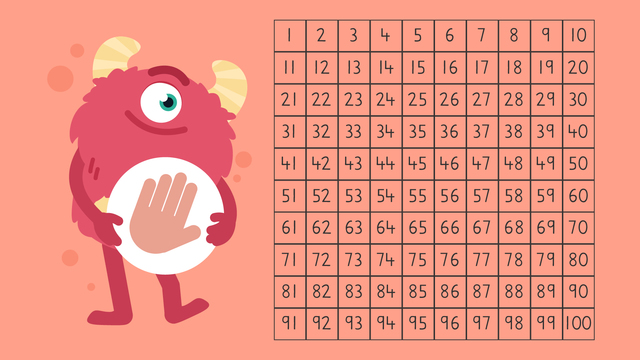# Adding Using a Chart — Let's PracticeRating

Ø 3.6 / 5 ratings
The authorsTeam Digital

## Basics on the topicAdding Using a Chart — Let's Practice

Let's practice learning about how to add using a chart with Razzi!

## Adding Using a Chart — Let's Practice exercise

Would you like to apply the knowledge you’ve learned? You can review and practice it with the tasks for the video Adding Using a Chart — Let's Practice.
• ### Help Razzi add using the number chart.

Hints

Start by identifying the larger addend or number, 12 on the chart.

Count up the second addend or number, 4 to find the solution.

Solution

• Start by identifying the larger addend or number, 12 on the chart.
• Count up the second addend or number, 4 to find the solution.
12 + 4 = 16

• ### Razzi needs to count blocks.

Hints

Which is the larger addend or number on the chart?

Count up the second addend or number to find the solution.

Solution

• Start by identifying the larger addend or number, 18 on the chart.
• Count up the second addend or number, 7 to find the solution.
18 + 7 = 25

Hints

Start by identifying the larger addend or number on the chart.

Count up the second addend or number to find the solution.

Solution

22 + 5 = 27

• Start by identifying the larger addend or number, 22 on the chart.
• Count up the second addend or number, 5 to find the solution.
37 + 3 = 40
• Start by identifying the larger addend or number, 37 on the chart.
• Count up the second addend or number, 3 to find the solution.
56 + 6 = 62
• Start by identifying the larger addend or number, 56 on the chart.
• Count up the second addend or number, 6 to find the solution.

Hints

Which is the larger number?

How much should you count up by?

Solution

67 + 8 = 75

• Start by identifying the larger addend or number, 67 on the chart.
• Count up the second addend or number, 8 to find the solution.
45 + 5 = 50
• Start by identifying the larger addend or number, 45 on the chart.
• Count up the second addend or number, 5 to find the solution.
72 + 9 = 81
• Start by identifying the larger addend or number, 72 on the chart.
• Count up the second addend or number, 9 to find the solution.

• ### What are the steps for adding using a number chart?

Hints

Do you identify the larger or smaller addend first?

Do you count up or back the second addend?

Solution

Steps for adding using a number chart:

• Start by identifying the larger addend on the chart.
• Count up the second addend.
• Find the solution.

• ### Razzi has a two part problem to solve.

Hints

Start at the larger number and count up the second number.

Count up the third number to find the solution.

Solution

27 + 5 + 7 = 39

• Start by identifying the larger addend or number, 27 on the chart.
• Count up the second addend or number, 5 to get 32.
• Count up the third number, 7 to find the solution.